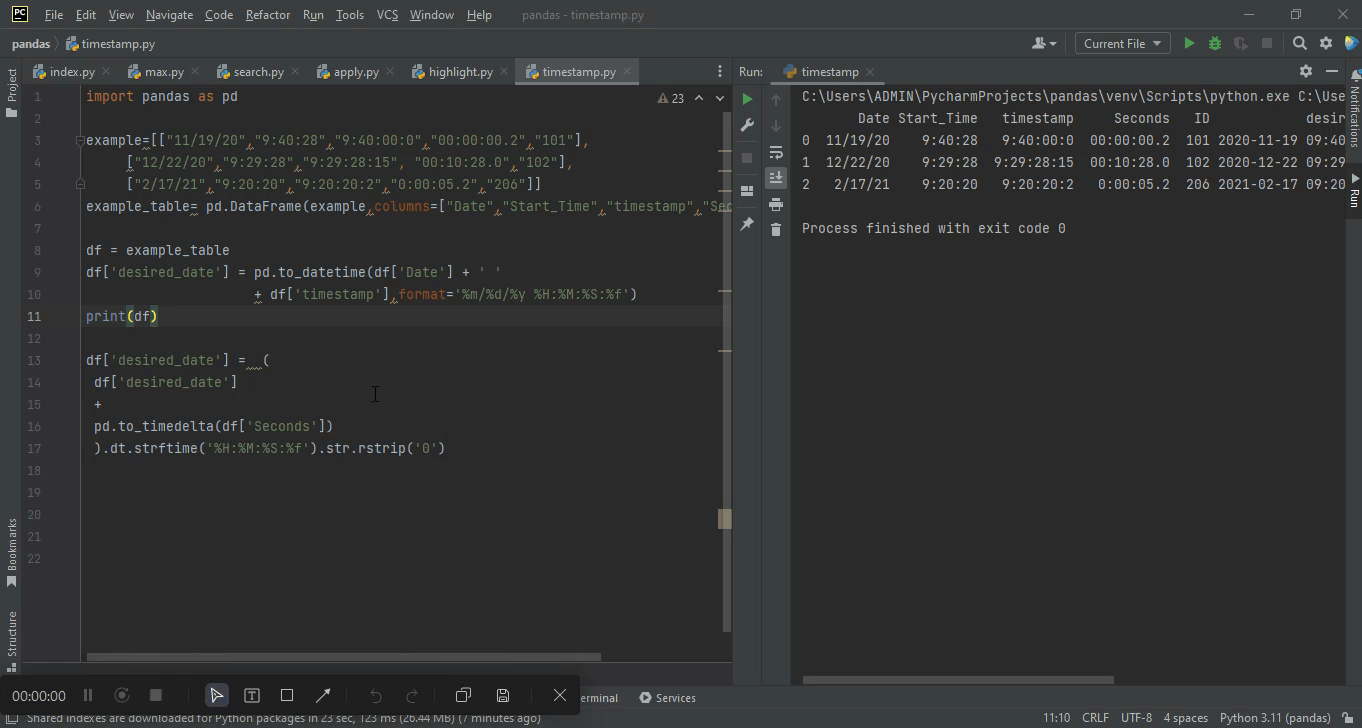# Adding and subtracting the second column from the timestamp column using pandasby vsasikalabeUpdated: Apr 6, 2023Solution Kit

This code helps work with time data in a Pandas DataFrame by providing a way to add and subtract time durations from timestamp data. This is useful for various calculations and analyses involving time data, such as calculating the duration and time between events or creating time-based plots and visualizations. The code also uses pandas functions and methods such as pd.to_timedeltadt.strftime, and str.rstrip to manipulate and format time data in a Pandas DataFrame. The code can streamline and simplify time-based data analysis tasks in Python by providing this functionality.

These are several Pandas and Python functions and methods used in the code snippet provided:

• pd.to_timedelta: This function converts a string or numerical value representing a time duration into a pandas Timedelta object. In the code, it is used to convert the values in the "Seconds" column into Timedelta objects.
• dt.strftime: This method formats a datetime object as a string with a specified format string. The code is used to format the final datetime object as a string with the format '%H:%M:%S:%f'.
• str.rstrip: This method removes trailing characters from a string. In the code, it is used to remove any trailing zeros from the resulting string format of the datetime object.
• pd.Timestamp: This class represents a specific moment in time with high precision. In the code, it is used to convert the "desired_date" column, which combines the "Date" and "timestamp" columns into a datetime object, to a pandas Timestamp object.

This code provides a powerful tool for working with time data in a Pandas DataFrame. This process is useful when working with time data in a Pandas DataFrame because it allows you to manipulate it to perform various calculations and analyses. For example, you could use this code to calculate an event's end time by adding the event's duration to the start time. Alternatively, you could use this code to calculate the time elapsed between two events by subtracting the second event's start time from the first event's end time.

Here is an example of how to Add and subtract the second column from the timestamp column using pandas:Preview of the output that you will get on running this code from your IDE.

### Code

In this solution we used pandas library of python.

### Instructions

Follow the steps carefully to get the output easily.

2. Copy the snippet using the 'copy' and paste it in your IDE.
3. Add required dependencies and import them in Python file.
4. Run the file to generate the output.

I hope you found this useful. I have added the link to dependent libraries, version information in the following sections.

I found this code snippet by searching for '  Adding and subtracting seconds column from timestamp column using pandas 'in kandi. You can try any such use case!

### Environment Tested

I tested this solution in the following versions. Be mindful of changes when working with other versions.

1. The solution is created in PyCharm 2022.3.
2. The solution is tested on Python 3.11.1
3. Pandas version-1.5.2.

Using this solution, we are able to add and subtract seconds column from timestamp column using pandas with simple steps. This process also facilities an easy way to use, hassle-free method to create a hands-on working version of code which would help us to add and subtract seconds column from timestamp column using pandas.

### Dependent Library

pandasby pandas-dev

Python38689Version:v2.0.2Flexible and powerful data analysis / manipulation library for Python, providing labeled data structures similar to R data.frame objects, statistical functions, and much more

Support
Quality
Security
Reuse

pandasby pandas-dev

Python38689Version:v2.0.2License: Permissive (BSD-3-Clause)

Flexible and powerful data analysis / manipulation library for Python, providing labeled data structures similar to R data.frame objects, statistical functions, and much more
Support
Quality
Security